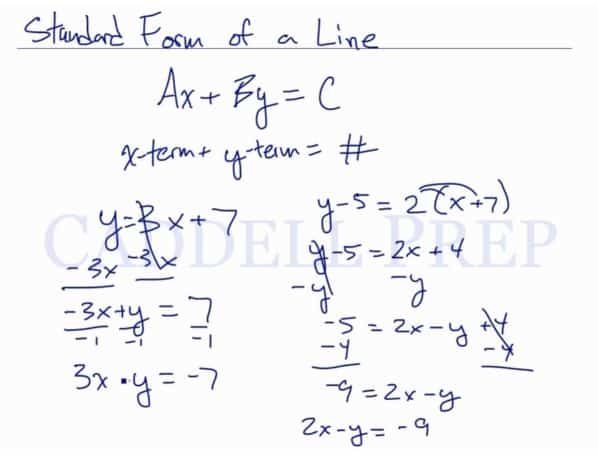This video explains how to convert linear functions from slope-intercept form and point-slope form, to the standard form of the equation of a line. After you finish this lesson, view all of our Algebra 1 lessons and practice problems.

The standard form of a line is written as:$Ax + By = C$

where A, B, and C, are constants.

An example would be:$y = 3x + 7$

Would be written as:$3x - y = -7$

Examples of Standard Form Of The Equation Of A Line

Example 1$2x=9-y$

The standard form of a line is written as:$Ax + By = C$

Let’s bring all the variables to one side by adding$y$ on both sides$2x+y=9-y+y$
Therefore, we have:$2x+y=9$

Example 2$3(x+5)-2=20+y$
The standard form of a line is written as:$Ax + By = C$

First, distribute$3$ in each of the terms inside the parenthesis.$3x+15-2=20+y$$3x+13=20+y$

Let’s bring all the variables to one side by adding$y$ on both sides$3x+13-y=20+y-y$$3x+13-y=20$

Then bring all the constant on one side by subtracting 13 on both sides$3x+13-y-13=20-13$

Therefore, we have:$3x-y=-7$

Video-Lesson Transcript

The standard form of a line is$Ax + By = C$

Where$(x-term) + (y-term) = \#$.

For example:$y = 3x + 7$

Let’s solve to make this look like this$(x-term) + (y-term) = \#$

Let’s get all the terms on one side first by subtracting$3x$ on both sides$y = 3x + 7$$-3x + y = 3x - 3x + 7$$-3x + y = 7$

It looks like it but we want the$x$ term to be positive. So we divide both sides by$-1$$\dfrac{-3x}{-1} + \dfrac{y}{-1} = \dfrac{7}{-1}$$3x - y = -7$

Let’s solve another one$y - 5 = 2 (x + 7)$$y - 5 = 2x + 14$

Let’s bring all the variables to one side by subtracting$y$ on both sides.$- 5 = 2x - y + 4$

Then bring all the constant on one side by subtracting$4$$- 5 - 4 = 2x - y + 4 - 4$$- 9 = 2x - y$

Let’s just rewrite it$2x - y = -9$

Just to recap it’s just$(x-term) + (y-term) = \#$.